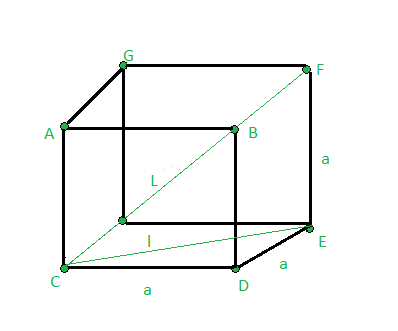# Find the diagonal of the Cube

Given a side of the cube a. The task is to find the length of the diagonal of the cube.Examples:

Input : a = 3
Output : 5.19615

Input : a = 6
Output : 10.3923

## Recommended: Please try your approach on {IDE} first, before moving on to the solution.

Formula :

Length of diagonal of the cube = sqrt(3) * side

Proof :

Use Pythagorean Theorem,
In triangle CED,
CE2 = CD2 + DE2
l2 = a2 + a2 ———>(1)
In triangle CFE,
CF2 = CE2 + EF2
L2 = l2 + a2
use l2 value from equation(1),
L2 = a2 + a2 + a2
= 3*a2
L = sqrt(3) * a

## C++

 `// CPP program to find length  ` `// of the diagonal of the cube  ` `#include ` `using` `namespace` `std; ` ` `  `// Function to find length  ` `// of diagonal of cube ` `float` `diagonal_length(``float` `a) ` `{ ` `    ``float` `L; ` ` `  `    ``// Formula to Find length  ` `    ``// of diagonal of cube ` `    ``L = a * ``sqrt``(3); ` ` `  `    ``return` `L; ` `} ` ` `  `// Driver code ` `int` `main() ` `{ ` ` `  `    ``float` `a = 5; ` ` `  `    ``// Function call ` `    ``cout << diagonal_length(a); ` ` `  `    ``return` `0; ` `} `

## Java

 `// Java program to find length  ` `// of the diagonal of the cube ` `class` `GFG  ` `{ ` `     `  `    ``// Function to find length  ` `    ``// of diagonal of cube ` `    ``static` `float` `diagonal_length(``float` `a)  ` `    ``{ ` `        ``float` `L; ` `         `  `        ``// Formula to Find length  ` `        ``// of diagonal of cube ` `        ``L = a * (``float``)Math.sqrt(``3``); ` `         `  `        ``return` `L; ` `    ``} ` `     `  `    ``// Driver Code ` `    ``public` `static` `void` `main (String[] args) ` `    ``{ ` `        ``float` `a = ``5``; ` `         `  `        ``// Function call ` `        ``System.out.println(diagonal_length(a)); ` `    ``} ` `} ` ` `  `// This code is contributed by ` `// sanjeev2552 `

## Python3

 `# Python3 program to find length ` `# of the diagonal of the cube ` `from` `math ``import` `sqrt ` ` `  `# Function to find length ` `# of diagonal of cube ` `def` `diagonal_length(a): ` `    ``L ``=` `0` ` `  `    ``# Formula to Find length ` `    ``# of diagonal of cube ` `    ``L ``=` `a ``*` `sqrt(``3``) ` ` `  `    ``return` `L ` ` `  `# Driver code ` `a ``=` `5` ` `  `# Function call ` `print``(diagonal_length(a)) ` ` `  `# This code is contributed by Mohit Kumar `

## C#

 `// C# program to find length  ` `// of the diagonal of the cube  ` `using` `System; ` `class` `GFG ` `{ ` `// Function to find length  ` `// of diagonal of cube ` `static` `float` `diagonal_length(``float` `a) ` `{ ` `    ``float` `L; ` ` `  `    ``// Formula to Find length  ` `    ``// of diagonal of cube ` `    ``L = a * (``float``)Math.Sqrt(3); ` ` `  `    ``return` `L; ` `} ` ` `  `// Driver code ` `public` `static` `void` `Main() ` `{ ` `    ``float` `a = 5; ` ` `  `    ``// Function call ` `    ``Console.Write(diagonal_length(a)); ` `} ` `} ` ` `  `// This code is contributed by Nidhi `

## PHP

 ` `

Output:

```8.66025
```

Attention reader! Don’t stop learning now. Get hold of all the important DSA concepts with the DSA Self Paced Course at a student-friendly price and become industry ready.

My Personal Notes arrow_drop_upCheck out this Author's contributed articles.

If you like GeeksforGeeks and would like to contribute, you can also write an article using contribute.geeksforgeeks.org or mail your article to contribute@geeksforgeeks.org. See your article appearing on the GeeksforGeeks main page and help other Geeks.

Please Improve this article if you find anything incorrect by clicking on the "Improve Article" button below.

Article Tags :
Practice Tags :

Be the First to upvote.

Please write to us at contribute@geeksforgeeks.org to report any issue with the above content.